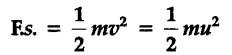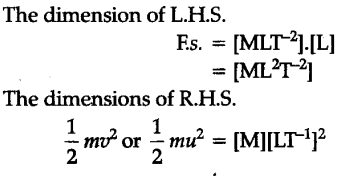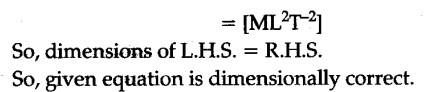# Whether equation is dimensionally correct, where m is mass of the body, v is its final velocity, u is its initial velocity, F is applied force and s is distance covered

Whether equationis dimensionally correct, where m is mass of the body, v is its final velocity, u is its initial velocity, F is applied force and s is distance covered.